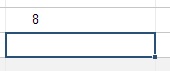# Create and Edit Formulas in Smartsheet

Smartsheet
• Pro
• Enterprise

## Capabilities

### Who can use this capability

The sheet Owner and Admins can create and edit formulas in locked and unlocked fields. The Owner, Admins, and Editors can create and edit formulas in unlocked fields.

Use formulas to calculate numeric values or automate aspects of your sheet. You can create formulas, for example, to sum a range of expenses, or to check a box when a specific value is entered in a field.

### Create a formula

You can create a formula in the following field types:

• Fields not being used for dependencies or resource management. For more information, see Enable Dependencies and Use Predecessors.
• Text/Number (as long as they're not being used in dependencies or resource management)
• Contact List fields (as long as they're not being used in resource management)
• Date (as long as they're not being used in dependencies or resource management)
• Dropdown List
• Checkbox
• Symbol
• % Allocation

To place a formula in a field:

1. Select the desired field.
2. Type an equal sign in the field, and then type the calculation.3. After you’ve typed in your formula, press [Enter].The result is displayed in the field containing the formula.

Formulas can be placed in Contact List fields. Automatically assign people to tasks with a VLOOKUP formula, for example. Note that formulas can’t be placed in Contact List fields being used for resource management.

#### Functions

Formulas can include functions, such as SUM, AVG, or IF. Functions perform calculations with or manipulate data in sheets. For the complete list of functions available in Smartsheet, see the Smartsheet Functions List.

#### Formula operators

You can use the following operators in Smartsheet formulas.

 Symbol Description + Add - Subtract * Multiply / Divide ^ Exponent < Less than > Greater than >= Greater than or equal to <= Less than or equal to = Equal to <> Not equal to

### Edit an existing formula

To edit an existing formula:

1. Double-click a field containing the formula to open it for editing.
2. Make your desired formula changes, and then press Enter.

If you change your mind about editing the formula, press Esc to exit edit mode and revert to the pre-edited formula.

### Tips for easier formula creation

#### Use column formulas

When you need a formula applied consistently and uniformly to an entire column, column formulas are the perfect solution. Build your formula in a cell, and quickly convert it to apply to every cell in the column. Regardless of how new rows get inserted into the sheet, the column formula will automatically apply. For more information, see Set Formulas for All Rows with Column Formulas.

#### Use the sum tool

If you're not sure which function to use, you can click the down arrow on the right of the Sum button in the toolbar and select a function.

The Sum button will attempt to provide you with a formula based on the field you have selected in the sheet and any hierarchical relationships with the selected field. For example, selecting the field of a parent row, then clicking the Sum icon will produce a =SUM(CHILDREN()) formula in the field.

After you create a formula, you can modify it at any time by double-clicking in the selected field or by pressing F2 (fn + F2 on a Mac).

#### Work with percentages

Smartsheet treats percentages as values between 0 and 1. When you create formulas in columns formatted for percent (using thePercentage Format button in the toolbar), use decimal values. For example...

=0.5 + 0.4

...will return 90% in a column formatted for percentage...

=5 + 4

...will return 900% in a column formatted for percentage.

#### Copy a formula with drag-fill or auto-fill

If you have a formula that you’d like to use in multiple fields without having to manually type the formula in each field, use the following methods to quickly copy the formula to other areas of your sheet:

• Drag-fill—You can drag from the lower-right corner of a selected field to copy a formula across contiguous fields in the sheet. As you copy, the formula will automatically change its respective field references.
• Auto-fill—You can have Smartsheet automatically copy a formula to new, vertically adjacent fields that enter the sheet. When auto-filled, the new formula will automatically change its respective field references.

### Areas where formula use is restricted

These fields can't contain formulas:

• Contact list fields that are being used in resource management
• System fields (for example, Modified By)
• Default columns (attachments, comments, row action indicator)
• The following fields being used for dependencies and resource management:
• Start Date
• End Date
• Duration
• Predecessors
• % Complete

You can't create formulas in these features:

• Forms
• Update requests (Formula results can still be viewed in update requests, but they can't contain standalone formulas.)
• Reports (Formula results can still be viewed in reports, but they can't contain standalone formulas.)
• Dashboards (Formula results can be viewed in dashboards, but dashboards can't contain standalone formulas.)

Formulas can't be edited from the following features in Smartsheet:

• Forms
• Update requests (Formula results can still be viewed in update requests, but the formulas can't be edited.)
• Reports (Formula results can still be viewed in reports, but the formulas can't be edited.)
• Dashboards (Formula results can be viewed in dashboards, but the formulas can't be edited.)

#### Still need help?

Use the Formula Handbook template to find more support resources, and view 100+ formulas, including a glossary of every function that you can practice working with in real time, and examples of commonly used and advanced formulas.

Find examples of how other Smartsheet customers use this function or ask about your specific use case in the Smartsheet online Community.